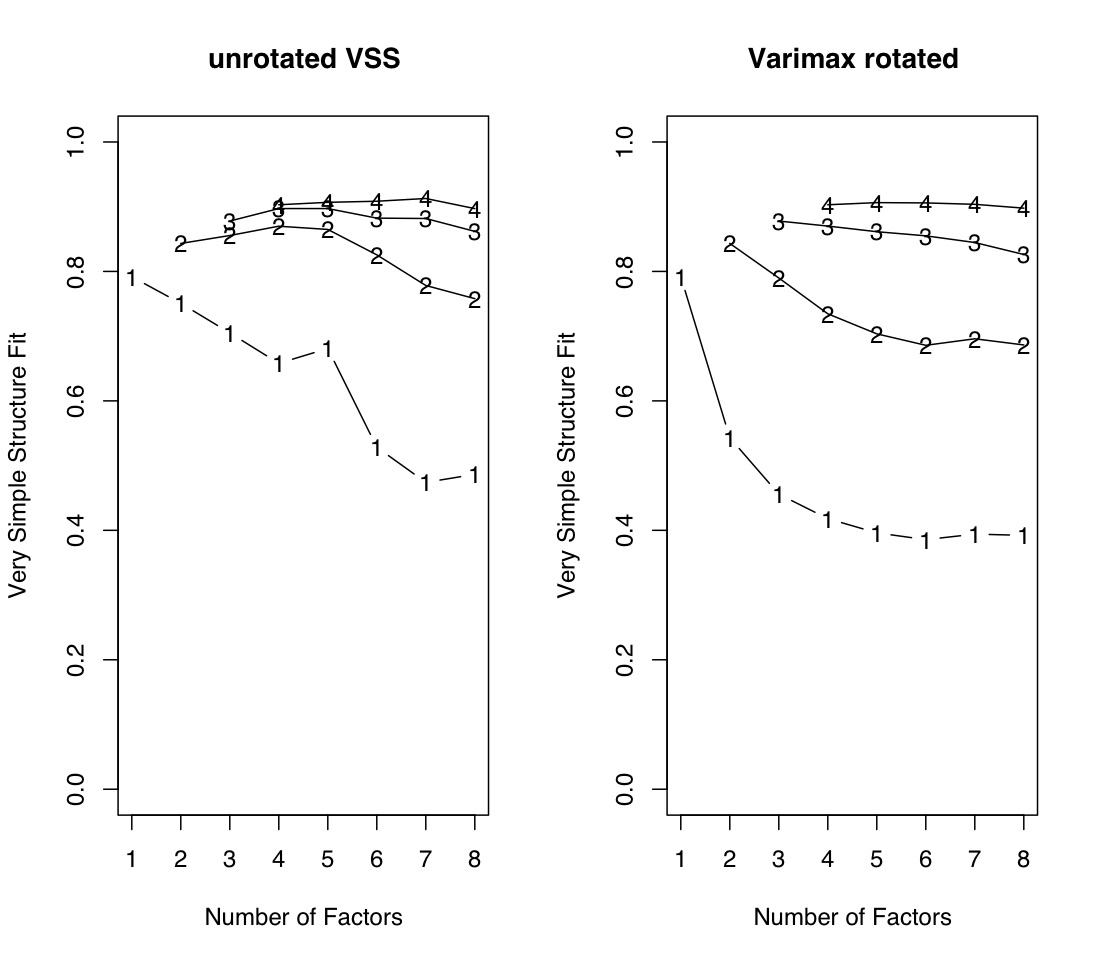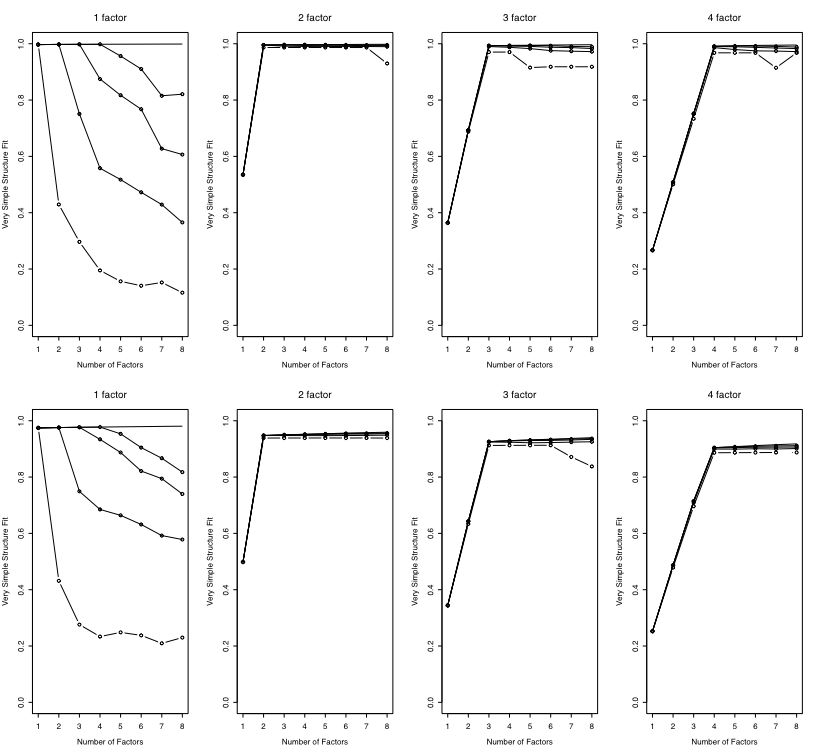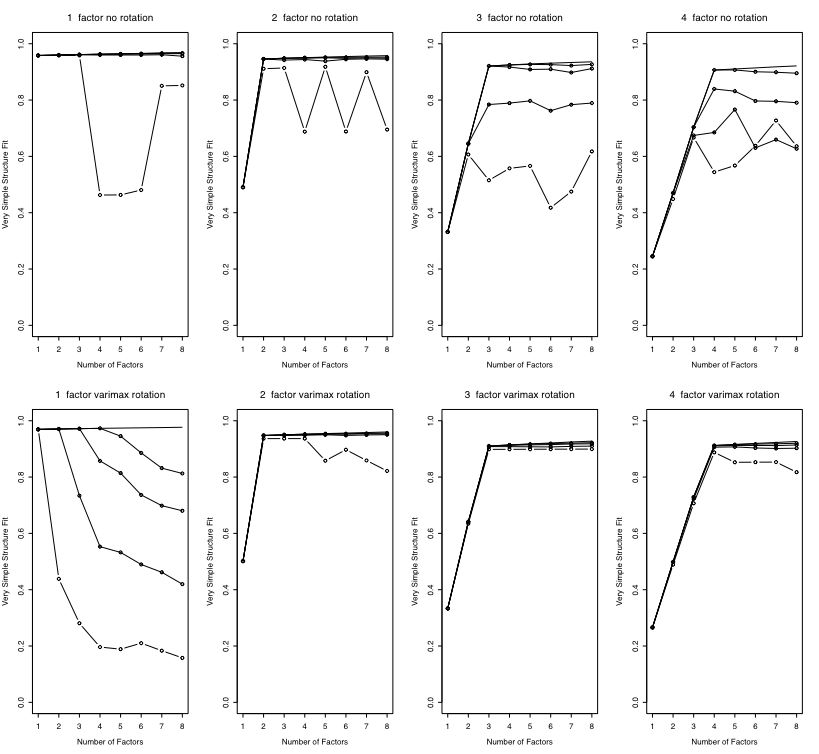The classic factor model is that elements of a correlation matrix (R) may be approximated by the product of a factor matrix (F) times the transpose of F (F') plus a diagonal matrix of uniquenesses: R ~ FF' + U². Factor analysis is a fundamental part of scale development and data simplification in many fields.

Determining the most interpretable number of factors from a factor analysis is perhaps one of the greatest challenges in factor analysis. There are many solutions to this problem, none of which is uniformly the best. "Solving the number of factors problem is easy, I do it everyday before breakfast. But knowing the right solution is harder" (Kaiser, 195x).

Techniques most commonly used include

1. Extracting factors until the chi square of the residual matrix is not significant.
2. Extracting factors until the change in chi square from factor n to factor n+1 is not significant.
3. Extracting factors until the eigen values of the real data are less than the corresponding eigen values of a random data set of the same size (parallel analysis).
4. Plotting the magnitude of the successive eigen values and applying the scree test (a sudden drop in eigen values analogous to the change in slope seen when scrambling up the talus slope of a mountain and approaching the rock face.
5. Extracting principal components until the eigen value <1.
6. Extracting factors as long as they are interpetable.
7. Using the Very Structure Criterion.

Each of the procedures has its advantages and disadvantages. Using either the chi square test or the change in square test is, of course, sensitive to the number of subjects and leads to the nonsensical condition that if one wants to find many factors, one simlpy runs more subjects. Parallel analysis is partially sensitive to sample size in that for large samples the eigen values of random factors will be very small. The scree test is quite appealling but can lead to differences of interpretation as to when the scree "breaks". The eigen value of 1 rule, although the default for many programs, seems to be a rough way of dividing the number of variables by 3. Extracting interpretable factors means that the number of factors reflects the investigators creativity more than the data. VSS, while very simple to understand, will not work very well if the data are very factorially complex. (Simulations suggests it will work fine if the complexities of some of the items are no more than 2).

Most users of factor analysis tend to interpret factor output by focusing their attention on the largest loadings for every variable and ignoring the smaller ones. Very Simple Structure operationalizes this tendency by comparing the original correlation matrix to that reproduced by a simplified version (S) of the original factor matrix (F). R ~ SS' + U². S is composed of just the c greatest (in absolute value) loadings for each variable. C (or complexity) is a parameter of the model and may vary from 1 to the number of factors.

The VSS criterion compares the fit of the simplified model to the original correlations: VSS = 1 -sumsquares(r*)/sumsquares(r) where R* is the residual matrix R* = R - SS' and r* and r are the elements of R* and R respectively.

VSS for a given complexity will tend to peak at the optimal (most interpretable) number of factors (Revelle and Rocklin, 1979).

Although originally written in Fortran for main frame computers, VSS has been adapted to micro computers (e.g., Macintosh OS 6-9) using Pascal. With the availability of an extremely powerful public domain statistical package R, we now release R code for calculating VSS. This is a preliminary release, with further modifications to make it a package within the R system. For an introduction in R for psychologists, see tutorials by Baron and Li or Revelle.

To use this code within R, simply copy the following commands into R and then call the VSS function. (Even better, install the "psych" package from the personality-project.

## Examples

The first example uses the Harman 24 variable problem (see also the ICLUST example), and compares a default run with a varimax rotation.

```
r.mat<- Harman74.cor\$cov
iq.vss <- VSS(r.mat)
VSS.plot(iq.vss)   #see graphic window
quartz()      #make a new window so we can compare the two
iq.vss.v <- VSS(r.mat,rotate="varimax")
VSS.plot(iq.vss.v)

#alternatively, draw two graphs on one page:
par(mfrow=c(1,2))
VSS.plot(iq.vss,plottitle="unrotated VSS")   #see graphic window
VSS.plot(iq.vss.v,plottitle="Varimax rotated")

```## VSS source code

The following can be copied into R directly, although using the psych package is more convenient. In addition, the package and the associated help windows are maintained with the most up to date code. This document should not be seen as the definitive version.

```#Very Simple Structure
#a method for determining the most interpretable number of factors to extract
#written as a function library  to allow for easier use (eventually)
#see Revelle and Rocklin, 1979 for basic algorithm and justification of program
#makes repeated calls to the factor analysis package (factanal) of R
#parameter calls to VSS are similar to those of factanal

#things to do: add feature allowing for VSS applied to principal components
#add feature to allow doing VSS on specified correlation and factor matrices

"VSS" <-
function (x,n=8,rotate="none",diagonal=FALSE,pc=FALSE,n.obs=1000,...)     #apply the Very Simple Structure Criterion for up to n factors on data set x
#x is a data matrix
#n is the maximum number of factors to extract  (default is 8)
#rotate is a string "none" or "varimax" for type of rotation (default is "none"
#diagonal is a boolean value for whether or not we should count the diagonal  (default=FALSE)
# ... other parameters for factanal may be passed as well
#e.g., to do VSS on a covariance/correlation matrix with up to 8 factors and 3000 cases:
#VSS(covmat=msqcovar,n=8,rotate="none",n.obs=3000)

{             #start Function definition
#first some preliminary functions

{  n=length(x)          	#how many columns in this row?
temp <- x                #make a temporary copy of the row
x <- rep(0,n)            #zero out x
for (j in 1:c)
{
locmax <- which.max(abs(temp))                     #where is the maximum (absolute) value
x[locmax] <- sign(temp[locmax])*max(abs(temp))    #store it in x
temp[locmax] <- 0                                  #remove this value from the temp copy
}
return(x)                                             #return the simplified (of complexity c) row
}

complexmat <- function(x,c)           #do it for every row   (could tapply somehow?)
{
nrows <- dim(x)
ncols <- dim(x)
for (i in 1:nrows)
return(x)
}

#now do the main Very Simple Structure  routine

complexfit <- array(0,dim=c(n,n))        #store these separately for complex fits
complexresid <-  array(0,dim=c(n,n))

vss.df <- data.frame(dof=rep(0,n),chisq=0,prob=0,sqresid=0,fit=0) #keep the basic results here
for (i in 1:n)                            #loop through 1 to the number of factors requested
{
if(!pc) {
if (dim(x) ==dim(x)) {f <- factanal(x,i,rotation=rotate,covmat=x,n.obs=n.obs,...) } else {
f <- factanal(x,i,rotation=rotate,...)}  #do a factor analysis with i factors and the rotations specified in the VSS call
if (i==1)
{original <- f\$correlation         #just find this stuff once
sqoriginal <- original*original    #squared correlations
totaloriginal <- sum(sqoriginal) - diagonal*sum(diag(sqoriginal) )   #sum of squared correlations - the diagonal
}
} else {f <- principal(x,i)
if (i==1)
{original <- x       #the input to pc is a correlation matrix, so we don't need to find it again
sqoriginal <- original*original    #squared correlations
totaloriginal <- sum(sqoriginal) - diagonal*sum(diag(sqoriginal) )   #sum of squared correlations - the diagonal
}
}

model <- load %*% t(load)                 #reproduce the correlation matrix by the factor law R=  FF'
residual <- original-model              #find the residual  R* = R - FF'
sqresid <- residual*residual            #square the residuals
totalresid <- sum(sqresid)- diagonal * sum(diag(sqresid) )      #sum squared residuals - the main diagonal
fit <- 1-totalresid/totaloriginal       #fit is 1-sumsquared residuals/sumsquared original     (of off diagonal elements

if (!pc) {
vss.df[i,1] <- f\$dof                   #degrees of freedom from the factor analysis
vss.df[i,2] <- f\$STATISTIC             #chi square from the factor analysis
vss.df[i,3] <- f\$PVAL                  #probability value of this complete solution
}
vss.df[i,4] <- totalresid              #residual given complete model
vss.df[i,5] <- fit                     #fit of complete model

#now  do complexities -- how many factors account for each item

for (c in 1:i)

{
model <- simpleload%*%t(simpleload)           #the model is now a simple structure version  R ? SS'
residual <- original- model                   #R* = R - SS'
sqresid <- residual*residual
totalsimple <- sum(sqresid) -diagonal * sum(diag(sqresid))    #default is to not count the diagonal
simplefit <- 1-totalsimple/totaloriginal
complexresid[i,c] <-totalsimple
complexfit[i,c] <- simplefit
}

}     #end of i loop for number of factors

vss.stats <- data.frame(vss.df,cfit=complexfit,cresidual=complexresid)
return(vss.stats)

}     #end of VSS function

#####################################################################
#function to plot the output of VSS
#a little crude, in that it only plots complexities 1-4

plotVSS <- function(x,plottitle="Very Simple Structure",line=TRUE)
{
n=dim(x)
symb=c(49,50,51,52)              #plotting sym
plot(x\$cfit.1,ylim=c(0,1),type="b",ylab="Very Simple Structure Fit",xlab="Number of Factors",pch=49)
if (line) lines(x\$fit)

title(main=plottitle)
x\$cfit.2<-x\$fit
x\$cfit.3<-x\$fit
x\$cfit.3<-x\$fit
x\$cfit.4<-x\$fit
x\$cfit.4<-x\$fit
x\$cfit.4<-x\$fit
lines(x\$cfit.2)
points(x\$cfit.2,pch=50)
lines(x\$cfit.3)
points(x\$cfit.3,pch=symb)
lines(x\$cfit.4)
points(x\$cfit.4,pch=symb)
}

```

Output from VSS is a data.frame with n (number of factors to try) rows and 21 elements:

"dof" degrees of freedom in the model for this number of factors
"chisq" chi square for the the model
"prob" probability of chi square
"sqresid" the sum of the squared residuals for the full (non simple) model
"fit" fit statistic for full model
"cfit.1" fit statistic for complexity 1 (VSS 1)
"cfit.2" fit statistic for complexity 2
"cfit.3" ...
"cfit.4"
"cfit.5"
"cfit.6"
"cfit.7"
"cfit.8"
"cresidual.1" sum squares of residual correlations for complexity 1
"cresidual.2"
"cresidual.3"
"cresidual.4"
"cresidual.5"
"cresidual.6"
"cresidual.7"
"cresidual.8"
This output does not need to be displayed, but can rather be plotted using plotVSS.

Additional functions to become part of a VSS package include functions to do parallel analyses and generate a simple structure for a particular number of factors and variables.

```
#######
#generates random data to do parallel analyses to check VSS against random data

VSS.parallel <- function(ncases,nvariables)       #function call
{
simdata=matrix(rnorm(ncases*nvariables),nrow=ncases,ncol=nvariables)    #make up simulated data
testsim <- VSS(simdata,8,"none")
return(testsim)
}

######################################################################################

#with nfactors

{
theta=matrix(rnorm(ncases*nfactors),nrow=ncases,ncol=nvariables)  #generates nfactor independent columns, repeated nvariable/nfactor times)
error=matrix(rnorm(ncases*nvariables),nrow=ncases,ncol=nvariables) #errors for all variables
return(items)
}

```

Demonstrations of these functions and the graphics they produce are

```# test out VSS with 1 - 4 factors
meanloading=.7               #this implies item * item intercorrelations of .5 within a factor, actually fairly high
ncases=400                   #typical sample size

par(mfrow=c(2,4))            #2 rows and 4 columns allow us to compare results

#this first set removes the diagonal values and fits the off diagonal elements

for (i in 1:4)
plotVSS(x,paste(i, " factor"),line=FALSE) }

#this set leaves the diagonal on and thus fits are worse

for (i in 1:4)
plotVSS(x,paste(i, " factor")) }

#these next 8 analyses compare varimax to no rotations
#it seems as if VSS better detects the number of factors with rotated solutions
par(mfrow=c(2,4))            #2 rows and 4 columns allow us to compare results

for (i in 1:4)
plotVSS(x,paste(i, " factor no rotation")) }

for (i in 1:4)
The first figure compares the fit with or without the main diagonal as a function of the number of factors in the data.The second figure shows the fit as function of number of simulated factors and whether varimax or no rotation was done.